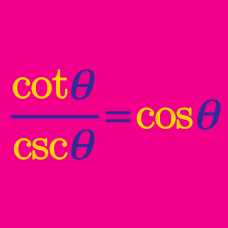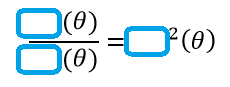Geometry

# Proving Trigonometric Identities Warmup

$\frac{\tan(\theta)}{\sin(\theta)}$ is equivalent to which of these?

If this equation is true...

$\sin^a(\theta) \cdot \csc^5(\theta) = \sin(\theta)$

...then what is $a$?

Simplify:

$\sin^2(\theta) \csc^3(\theta) \cos^4(\theta) \sec^5(\theta) \tan^6(\theta) \cot^{7}(\theta)$How many ways are there to fill the blanks so the equation is true, choosing from the six basic trigonometric functions (sin, cos, tan, csc, sec, cot)?

$\frac{\sin^2(\theta) + 1}{\sin(\theta)}$

Which expression is equivalent to the expression above?

×